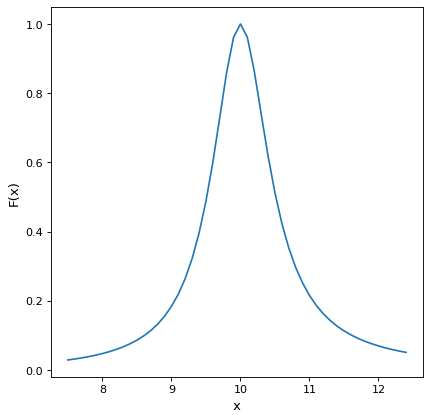# Drude1D¶

class astropy.modeling.physical_models.Drude1D(amplitude=1.0, x_0=1.0, fwhm=1.0, **kwargs)[source]

Drude model based one the behavior of electons in materials (esp. metals).

Parameters
amplitudefloat

Peak value

x_0float

Position of the peak

fwhmfloat

Full width at half maximum

Model formula:
$f(x) = A \frac{(fwhm/x_0)^2}{((x/x_0 - x_0/x)^2 + (fwhm/x_0)^2}$

Examples

import numpy as np
import matplotlib.pyplot as plt

from astropy.modeling.models import Drude1D

fig, ax = plt.subplots()

# generate the curves and plot them
x = np.arange(7.5 , 12.5 , 0.1)

dmodel = Drude1D(amplitude=1.0, fwhm=1.0, x_0=10.0)
ax.plot(x, dmodel(x))

ax.set_xlabel('x')
ax.set_ylabel('F(x)')

plt.show()


(png, svg, pdf)Attributes Summary

 amplitude fwhm input_units This property is used to indicate what units or sets of units the evaluate method expects, and returns a dictionary mapping inputs to units (or None if any units are accepted). param_names Names of the parameters that describe models of this type. return_units This property is used to indicate what units or sets of units the output of evaluate should be in, and returns a dictionary mapping outputs to units (or None if any units are accepted). x_0

Methods Summary

 evaluate(x, amplitude, x_0, fwhm) One dimensional Drude model function fit_deriv(x, amplitude, x_0, fwhm) Drude1D model function derivatives.

Attributes Documentation

amplitude = Parameter('amplitude', value=1.0)
fwhm = Parameter('fwhm', value=1.0)
input_units
param_names = ('amplitude', 'x_0', 'fwhm')

Names of the parameters that describe models of this type.

The parameters in this tuple are in the same order they should be passed in when initializing a model of a specific type. Some types of models, such as polynomial models, have a different number of parameters depending on some other property of the model, such as the degree.

When defining a custom model class the value of this attribute is automatically set by the Parameter attributes defined in the class body.

return_units
x_0 = Parameter('x_0', value=1.0)

Methods Documentation

static evaluate(x, amplitude, x_0, fwhm)[source]

One dimensional Drude model function

static fit_deriv(x, amplitude, x_0, fwhm)[source]

Drude1D model function derivatives.